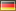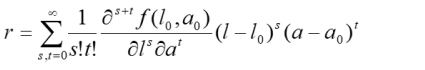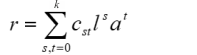## Site menu:## cNORM - Mathematical derivation

In our mathematical approach, the raw score r of a test scale is modeled as a continuous function of the latent person parameter l and an explanatory variable a.### Parameters

The person parameter l is the location of the person in relation to other persons in the reference group. This location can be expressed as a rank (= percentile) or as a standardized norm score. The latter is generated by a normal rank transformation from the percentile. The use of standardized norm scores is based in principle on the implicit assumption that the latent person parameter is normally distributed in the population. This applies to many, but not all, psychological or physiological parameters. For example, a physiological parameter such as the body mass index is distributed with positive skewness. Due to the similarity of the distribution functions, such quantities can nevertheless be modeled very well with the help of a normal rank transformation.

The explanatory variable a is a variable that covaries systematically with the quantity or ability to be measured. For example, the absolute reasoning ability increases monotonously in childhood and adolescence. In this case, the explanatory variable would be the age. Other abilities are more dependent on how long a child has been in school. The explanatory variable would then be the duration of schooling. Dichotomous variables (e.g. gender) can theoretically also be used as explanatory variables.

### Mathematical derivation

Although raw scores are integers in most psychometric tests, for the mathematical derivation a continuum must be assigned to these discrete scores. In this case, the considered function is smooth throughout the measuring range of the test, i.e., the function can be differentiated any number of times. Such functions can at any point P(l0, a0) be written as so-called Taylor series:Taylor series normally converge only within a finite radius around the point P against the function to be modeled. In practice, however, it has been shown that many functions (e.g., the data of psychological or physiological tests) can be approximated extremely well over a sufficient range.

Since the Taylor polynomial represents an infinite sum, this sum can only converge towards a finite value if the individual summands become tiny very quickly with increasing s and t. At the same time, this means that with a large s and t, the summands only make small contributions to the function value. The original function can therefore be approached with sufficient accuracy even if the sum is only built up to an index k instead of infinity. This index k is a smoothing factor. The higher k, the more likely it is that noise in the standardization sample is retraced in the model. To avoid over-fitting, do not select k higher than 5. As a rule of thumb, k = 4 will already lead to a very good approximation.

The approximation finally leads to a finite Taylor polynomial, which can be simplified even further. The faculties of s and t as well as the partial derivatives of the function with respect to l and a at point P(l0, a0) represent constants, just like l0 and a0 do. Multiplying the brackets therefore leads to the considerably simplified polynomial:The constants cst of this polynimial can be fitted by multiple regression on the basis of the manifest raw data.back to the overview